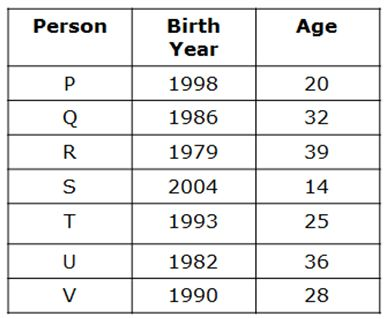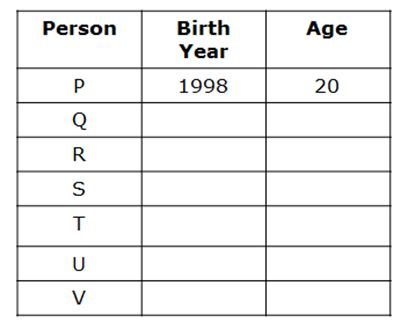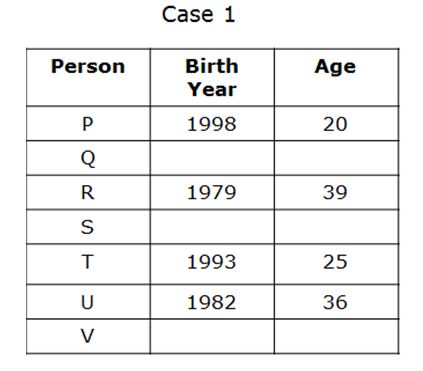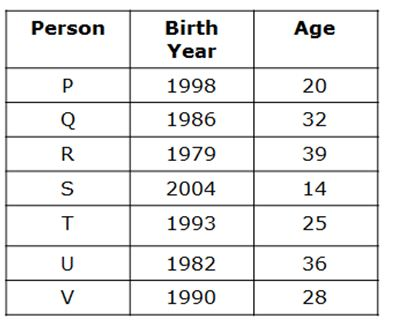# LIC AAO Mains Reasoning Questions 2019 (Day-05)

Dear Aspirants, Our IBPS Guide team is providing new series of Reasoning Questions for LIC AAO Mains 2019 so the aspirants can practice it on a daily basis. These questions are framed by our skilled experts after understanding your needs thoroughly. Aspirants can practice these new series questions daily to familiarize with the exact exam pattern and make your preparation effective.

[WpProQuiz 5865]

### Click Here for SBI PO Pre 2019 High-Quality Mocks Exactly on SBI Standard

Directions (1-5): Study the given information carefully and answer the following questions.

Seven persons P, Q, R, S, T, U and V are born in a month of March in different years. Their ages are calculated on the same month with respect to 2018.

Note: If it is mentioned that a person’s age is considered as the last two digits of another person’s birth year, then it will be at any sequence. For example, if the age of X is considered as last two digit of birth year of Y which is 1945; then the age of X might be either 45 or 54.

None of the persons were born before 1974 and after 2008.

P was born on 1998. The difference between the age of P and T is 5 years. R’s age is equal to the last two digit of birth year of T and his age is not an even number. U’s age is a square number between T’s age and R’s age. S’s age is half of the age of V’s age whose age is last two digit of U’s birth year. The sum of ages of P and S is two more than that of the age of Q.

1) What is the age of S?

a) None of these

b) 16 years

c) 14 years

d) 22 years

e) 38 years

2) Which of the following year is the birth year of U?

a) 1984

b) 1993

c) 2000

d) None of these

e) 1982

3) If W was born between R and U then which of the following can be the birth year of W?

a) 1980

b) None of these

c) 1990

d) 1982

e) 1985

4) What will be the sum of ages of Second youngest and Third eldest person in the group?

a) 55 years

b) 52 years

c) 60 years

d) 49 years

e) None of these

5) Which of the following statement is true?

a) U is younger than Q

b) T is three year elder than V

c) None of the options are true

d) S is not the youngest person

e) Number of person born after Q is same as before T

Directions (6 -10): Study the following information to answer the given questions:

% R 3 6 M @ 5 S B W 9 C \$ 12 T * 15 L K # H 4 Q D

Step 1 – Those Numbers which is immediately preceded by the symbol and immediately followed by an Alphabet are written from the right end in ascending order.

Step 2 – After completing the step -1, interchanging the Odd number with the previous element in the series to form the step -2

Step 3 – After completing the step – 2, Alphabet which is immediately preceded by a symbol are written between Sixth and Fifth element from the Right end in reverse alphabetical order from left to right.

Step 4 – After completing the step – 3, The first ten element from the left end is written after the last element in series in the reverse order(It means tenth element from the left end is written immediately after the last element and so on..,)

6) Which of the following element is seventh to the right of the element which is tenth from the left end in step-4?

a) \$

b) *

c) B

d) W

e) 9

7) If the alphabet which is immediately followed by a constant is changed to next letter in alphabet series in step-4, then how many vowels are present in the newly formed series?

a) One

b) Two

c) Three

d) Four

e) None

8) If all the symbols are removed from the step -3, then which of the following element will be in the middle of the series?

a) 4

b) T

c) K

d) C

e) S

9) How many numbers are immediately followed and immediately preceded by an alphabet in step -4?

a) One

b) Two

c) Three

d) None

e) More than three

10) What is will be the product of the number which is eleventh from the left in step-4 and the number which is Seventeenth from the right end in step-3?

a) 135

b) 75

c) 180

d) 48

e) 108

Directions (1-5):Note: None of the person born after 2008.

If it mentions the person age is considered as last two digits of the person Birth year, then it will be at any sequence. For example, X age is considered as last two digit of Y birth year-1936, and then X age is either 36 or 63.

• P was born on1998.• The difference between the age of P and T is 5 years.
• R’s age is equal to the last two digit of birth year of T and his age is not an even number.• U’s age is a square number between T’s age and R’s age.• S’s age is half of the age of V’s age whose age is last two digit of U’s birth year.
• The sum of ages of P and S is two more than age of Q.Directions (6 -10):

%  R  3  6  M  @  5  S  B  W  9  C  \$  12  T  *  15  L  K #   H  4  Q  D

Step 1: %  R  3  6  M  @  S  B  W  9  C  \$   T  *   L  K #   H  4  Q  D 15  12  5

Step 2: %  3 R  6  M  @  S  B    9  W  C  \$   T  *   L  K #   H  4  Q  15 D   5 12

Step 3: %  3 R  6  M  @ B    9  W  C  \$    *   K #   4  T  S  L  H  Q  15  D   5  12

Step 4 : \$  *  K  #   4   T   S   L  H  Q  15  D  5  12  C  W  9  B  @  M  6  R  3%

Step 4 : \$  *  K  #   4   T   S   L  H  Q  15  D  5  12  C  W  9  B  @  M  6  R  3%

Alphabet which is immediately followed by a constant

T, S, L, H, C

By changing to next letter, we get

U, T, M, I, D – There are two vowels in the newly formed series.

3 R  6  M   B    9  W  C    K    4  T  S  L  H  Q  15  D   5  12